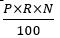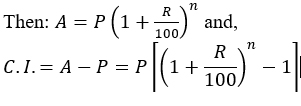# CBSE Worksheet for Chapter - 8 S.I and C.I Class 8

## Find CBSE Worksheet for chapter-8 S.I and C.I class 8

CLASS-8

BOARD: CBSE

Mathematic Worksheet - 8

TOPIC: S.I and C.I

For otherCBSE Worksheet for class 8 Mathematic check out main page of Physics Wallah.

#### SUMMARY

If S.I. is Simple Interest, P is Principle, A is Amount, R% is rate of interest and T is time.

Then:If A is the amount, P is the principle; R% is the rate of interest compounded annually and n is number of intervals,#### OBJECTI​VE:

1. In what time will Rs 64000 amount to Rs 68921 at 5% per annum, interest being compounded half- yearly?

(A) 5/3

(B) 5/2

(C) 4/2

(D) 5/4

2. In the difference between the C.I. and S.I. at the end of 2 years is Rs 100, what is the principal? Rate is 5% per annum in both the cases. Assume same principal for both the cases.

(A) Rs 50,000

(B) Rs 40,000

(C) Rs 10,000

(D) None of these

3. The compound interest on Rs 5000 at 4% per annum for 2 years compounded annually is:

(A) Rs804

(B) Rs 708

(C) Rs 408

(D) Rs 5408

4. Find the compound interest on Rs 15625 for 9 months, at 16% per annum, compounded quarterly.

(A) Rs1250

(B) Rs 1651

(C) Rs 1951

(D) Rs 2651

5. Difference between C.I & S.I. in a certain amount at 10% per annum for 2 yrs compounded annually is Rs 282. Find the principal.

(A) Rs28210

(B) Rs 28120

(C) Rs 28200

(D) None

6. If an amount is kept at S.I., it earns an interest of Rs 600 in first 2 years but when kept at C.I., it earns an interest of Rs 660 in the same period. The rate of interest will be:

(A) 10%

(B) 20%

(C) 30%

(D) None of these

7. A bacteria reproduces at the rate of 50% in every 15 min. in how much time will it be 27/8  times itself?

(A) 105 min

(B) 45 min

(C) 75 min

(D) 50 min

8. The population of a town increases 20% annually. What is the population after two years if present population is 2500?

(A) 3250

(B) 3500

(C) 3600

(D) 4000

9. A sum of Rs 8000 at compound interest becomes double after 5 years. After 20 years it will become:

(A) Rs 80000

(B) Rs 96000

(C) Rs 128000

(D) Rs 160000

#### SUBJ​ECTIVE:

1. Find the rate of interest at which a sum becomes four times of itself in 15 years at S.I.

2. How long will it take a sum of money invested at 5% p.a. S.I. to increase its value by 40%?

3. In what time a sum of Rs 2700 amounts to Rs 3240 at a rate of at S.I.

4. Find the amount of Rs 8000 for 3 years, compounded annually at 5% per annum. Also, find the compound interest.

5. At what rate percent per annum will a sum of Rs 2000 amount to Rs 2205 in 2 years, compounded annually?

6. The present population of town is 25000. It grows at the rate of 4%, 5% and 8% during the first year, second year and third year respectively. Find its population after 3 years.

### Solutions: to worksheet-8 Topic- S.I and C.I

1) C

2) B

3) C

4) C

5) C

6) B

7) B

8) C

9) C

#### Subjective:

1) 20%

2) 8 years

3) 3 years

4) Rs 9261; Rs 1261

5) 5%

6) 29484